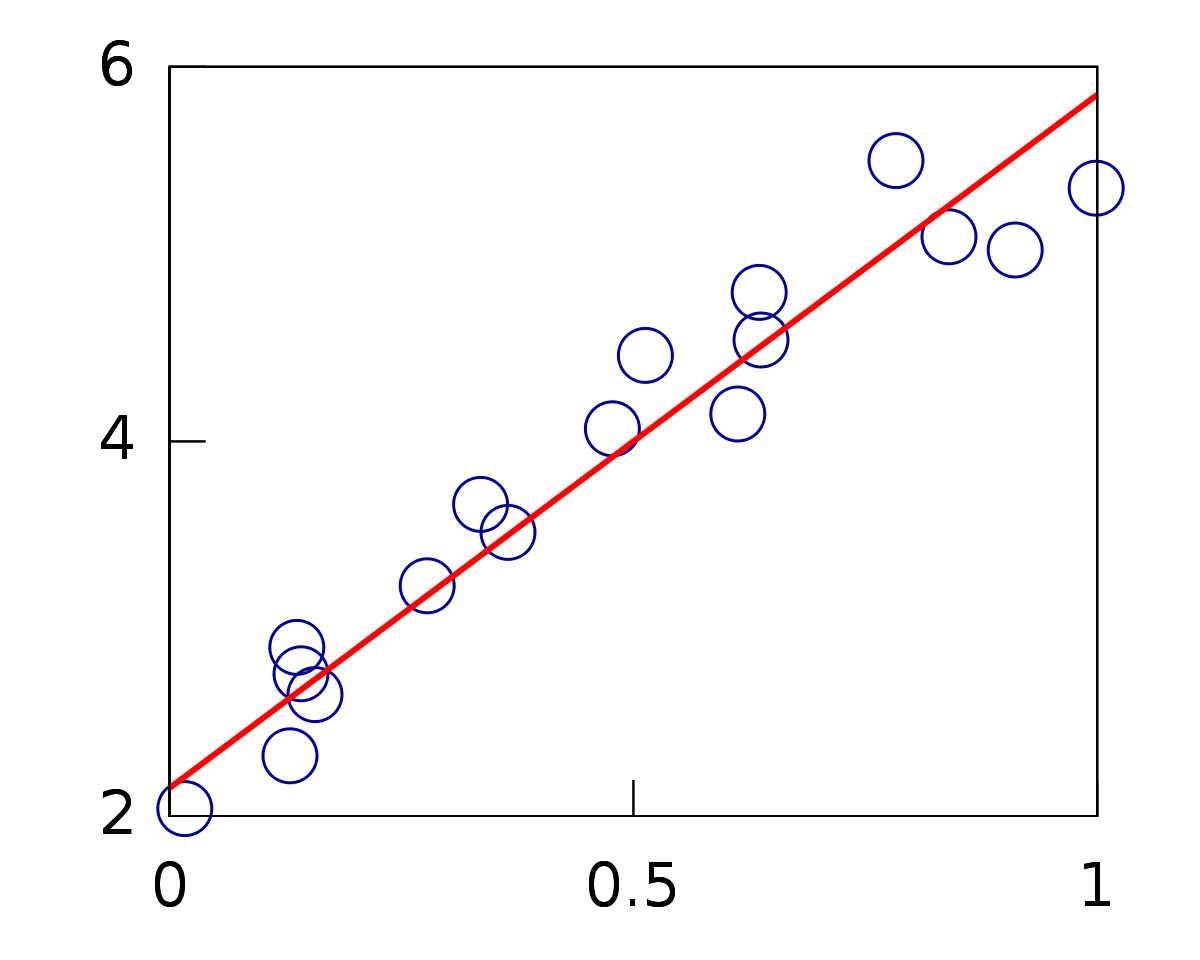# Perceiver AI Introduces Open Source GPU-based ElasticNet Regression Library in .NET# Perceiver AI Introduces Open Source GPU-based ElasticNet Regression Library in .NET

ElasticNet is a popular regression library (the most commonly used implementation can be found here) that, until recently, lacked a .NET implementation. That lack, coupled with the absence of a GPU-based library, significantly limited the scope of potential ElasticNet library users.

Now, thanks to Griffin Perry of Perceiver AI, who completed the alpha of a complete GPU-based ElectricNet regression library in C#/.NET, this is no longer the case.

The initial impetus for this project is that Perceiver AI’s core is undergoing a refactoring to further improve performance and to enable a myriad of new functionality.  This refactoring required a .NET implementation of the ElasticNet library.

ElasticNet is a form of regularized linear regression combining two commonly used penalties during training – the L1 and L2 penalty functions.

Regression modeling involves using selected input to predict a numeric value. Linear regression refers to an algorithm used for regression when a linear relationship is assumed between the target variable and the inputs used to determine that variable. Extensions can be added to linear regression in the form of penalties linked to the loss function in the training process, encouraging streamlined, less complex models with smaller coefficient values. When such extensions are used, the process is called either regularized linear regression or alternatively, penalized linear regression.

When a variable has a single input, this relationship takes the form of a line. When higher dimensions are involved, the relationship can be visualized as a hyperplane linking the input variables to the target variable. To find the coefficients of this model, optimization is used with a goal of minimizing the sum squared error that occurs between the projected and actual values of the expected targets.

An issue that can occur with linear regression modeling involves the occurrence of large estimated coefficients. Large coefficients can cause the model to become sensitive to inputs, potentially making it unstable. This can be exacerbated in the case of problems that feature a small number of observations, or samples, or ones that have a greater number of samples than input predictors or variables.

To handle the issue of instability with regression models, one technique is to add additional costs to the loss function for models with large coefficients. Linear regression models utilizing this approach are known as penalized linear regression models.

Two popular penalties used for this purpose are:

• L1 penalty: Penalizes a model with reference to the values of the sum of the absolute coefficient. This serves to reduce the size of all coefficients while also removing some predictors from the model by permitting the value of some coefficients to be reduced to zero.
• L2 penalty: Penalizes a model using the sum of the squared coefficient values. All coefficients are minimized in size by this method; however, no coefficients are removed from the model.

ElasticNet enables a balance between these two penalties, offering the opportunity for improved performance as compared with a model that only applies one of the two penalties to problems.

Griffin based the v1 algorithm powering the library on the ElasticNet regression from the open source library “smile.” As of the date of publication of this article, the software is in its alpha stage so please report or propose a fix for any issues found. The application uses the ILGPU library for its GPU integration, which allows the program to run on GPUs, greatly improving performance.

The ElasticNet regression library is published as an open source project from Perceiver AI. We are making the ElasticNet regression library freely available as a contribution to the open source community. We’d like to take this opportunity to invite the community to help improve the algorithm to make it more effective for all users – it’s located here.